# Difference between revisions of "Gröbner basis"

Gröbner bases are certain sets of multivariate polynomials with field coefficients. The importance of Gröbner bases stems from the fact that

i) many fundamental problems in algebraic geometry (commutative algebra, polynomial ideal theory) can be reduced by structurally easy algorithms to the construction of Gröbner bases; and

ii) there exists an algorithm by which for any given (finite) setof multivariate polynomials a Gröbner basisis constructed such thatandgenerate the same polynomial ideal. (This implies, in particular, that, conceived as algebraic sets of equations,andhave the same set of solutions.) Thus, Gröbner bases establish a uniform approach to the algorithmic solution of quite a few fundamental problems in algebraic geometry. New applications of Gröbner bases are discovered permanently. Also, the method of Gröbner bases has been carried over to domains other than polynomials over fields, e.g. polynomials with coefficients in certain rings, non-commutative polynomials, polynomial modules, and differential algebras. The algorithm that constructs Gröbner bases, various subalgorithms necessary for the construction of Gröbner bases, and various applications of Gröbner bases are routinely available in all modern mathematical software systems, as for example Mathematica and Maple.

A setof polynomials in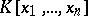, the polynomial ring over a fieldwith indeterminates, is called a Gröbner basis if and only if all polynomialsin the ideal generated bycan be reduced towith respect to.

This definition involves the notion of "reduction" , which can be conceived as a generalized division for multivariate polynomials. For example, letand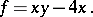Thencan be reduced, in one reduction step, with respect toby subtracting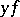from, yielding a new polynomial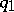:Note that, by the reduction, the power product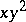occurring inis replaced by "smaller" power products. In fact, a large class of total orderings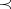on power products can be used in the Gröbner-bases approach for defining the notion of "smaller" . In the example, the polynomialcan be reduced further with respect toby subtracting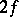, yielding a new polynomial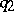:The polynomialcannot be reduced further with respect toand is called a reduced form ofwith respect to. A polynomialis reducible with respect to a setof polynomials if it is reducible with respect to some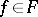.

Gröbner basescan be characterized in many other ways, for example by saying that the above reduction relation with respect tohas the Church–Rosser property, i.e. reduced forms are unique. The most important characterization of Gröbner bases is the content of the following main theorem:is a Gröbner basis if and only if, for all, the-polynomial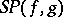can be reduced towith respect to.

The-polynomialof two polynomialsand(without loss of generality,andare taken to have leading coefficient) is defined as follows: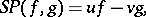where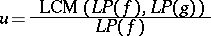and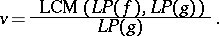Here,denotes the leading power product of the polynomial, i.e. the power product inwhich is maximal with respect to the total orderingand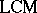denotes the least common multiple.

The above theorem is the crucial handle for constructing, for any given (finite) setof polynomials, a corresponding Gröbner basis, i.e. a Gröbner basisthat generates the same ideal as: One first checks whether the condition formulated in the main theorem holds for. If this is the case,is already a Gröbner basis. If, however, the reduction of any of the-polynomials yields a polynomial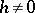, thenis added to the basis, new-polynomials must be considered, etc., until the algorithm terminates. Termination of this algorithm can be proved by an invocation of either Hilbert's basis theorem (cf. Hilbert theorem) or Dickson's lemma. By the above main theorem, whose proof is combinatorial, it is easy to see that the resulting basis is a Gröbner basis corresponding to the initial set.

Important applications of Gröbner bases are: exact and complete solution of algebraic systems of equations, analysis of algebraic varieties, computation of Hilbert functions and Hilbert polynomials, complete solution of linear Diophantine systems of equations with polynomial coefficients, effective computation in polynomial residue class rings, structural analysis of hypercomplex systems, automated geometrical theorem proving, implicitization of parameter representations of manifolds, solution of various problems in invariant theory and integer programming, and solution of word problems in commutative semi-groups.

The notion of a Gröbner basis, the main theorem, the algorithm based on the main theorem and some of the applications were introduced in [a2]. An easy-to-read summary of the theory is [a3]. The most comprehensive textbook on the subject is [a1].

How to Cite This Entry:
Gröbner basis. Encyclopedia of Mathematics. URL: http://encyclopediaofmath.org/index.php?title=Gr%C3%B6bner_basis&oldid=22529
This article was adapted from an original article by B. Buchberger (originator), which appeared in Encyclopedia of Mathematics - ISBN 1402006098. See original article